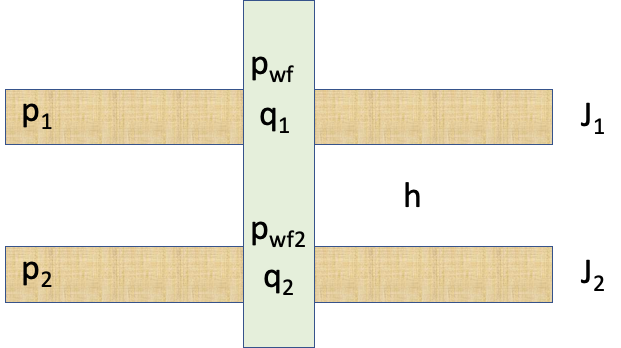# Dual-layer IPR with dynamic fracture

Consider a water injector with main pay in Reservoir Layer #1 and spontaneous fracture extending down to Reservoir Layer #2 (see Fig. 1).

Assume that fracture is not fixed and requires surplus pressure $//$ to get opened against the rock burden.

When injection bottomhole pressure $//$ is below fracture opening value $//$ then water is going to the main pay only (Reservoir Layer #1) and flow radially around the well.

When injection bottomhole pressure $//$ is above fracture opening value $// \Delta p_f\end{array} //]]>$ then water is going to the fracture and then gets distributed between Reservoir Layer #1 and Reservoir Layer 2Fig. 1. Dual-layer well schematic

 (1)
 (2)
 (3)
 (4)
 (5)
 (6)

where

Well

$//$

total subsurface flowrate of the well

$//$

total well productivity Index

$//$

apparent formation pressure of dual-layer formation

$//$

true vertical height between the layers tops

$//$

wellbore fuid density

$//$

gravity constant

$//$

fracture opening pressure
Layer #1

$//$

bottom-hole pr4essure at Layer #1 top

$//$

total subsurface flowrate of the Layer #1

$//$

formation pressure of the Layer #1

$//$

productivity Index of the Layer #1
Layer #2

$//$

bottom-hole pr4essure at Layer #2 top

$//$

total subsurface flowrate of the Layer #2

$//$

formation pressure of the Layer #2

$//$

productivity Index of the Layer #2

 (7)
 (8)

 (9)
 (10)

and

 (11)
 (12)

or

 (13)

or

 (14)